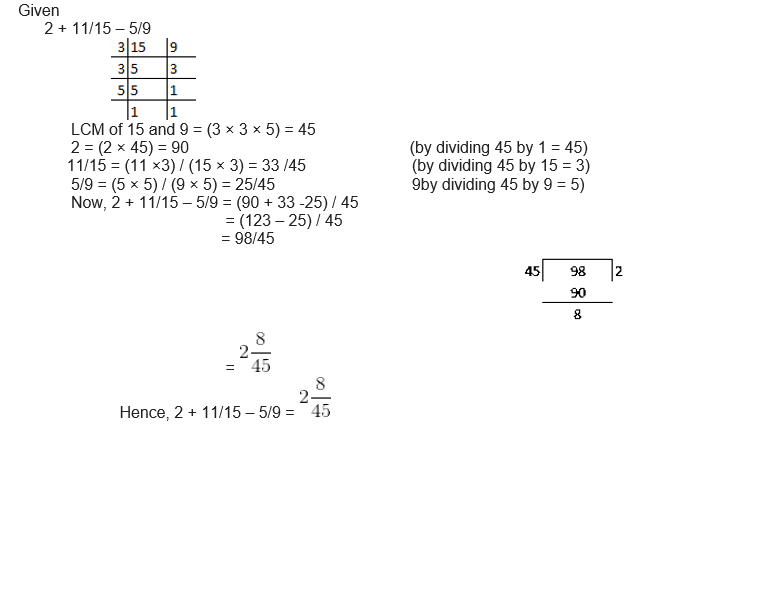# RS Aggarwal Solutions for Class 6 Maths Chapter 5 Fractions Exercise 5F

RS Aggarwal Solutions for Class 6 Maths Chapter 5 Exercise 5F are provided here in PDF. Exercise 5F contains the questions related to the topics like Subtraction of Like and Unlike Fractions. By practising problems using RS Aggarwal Solutions, students become experts in solving problems. The solutions PDF is a major source of study material for the students to perform well in the Maths exam.

## Download PDF of RS Aggarwal Solutions for Class 6 Maths Chapter 5 Fractions Exercise 5F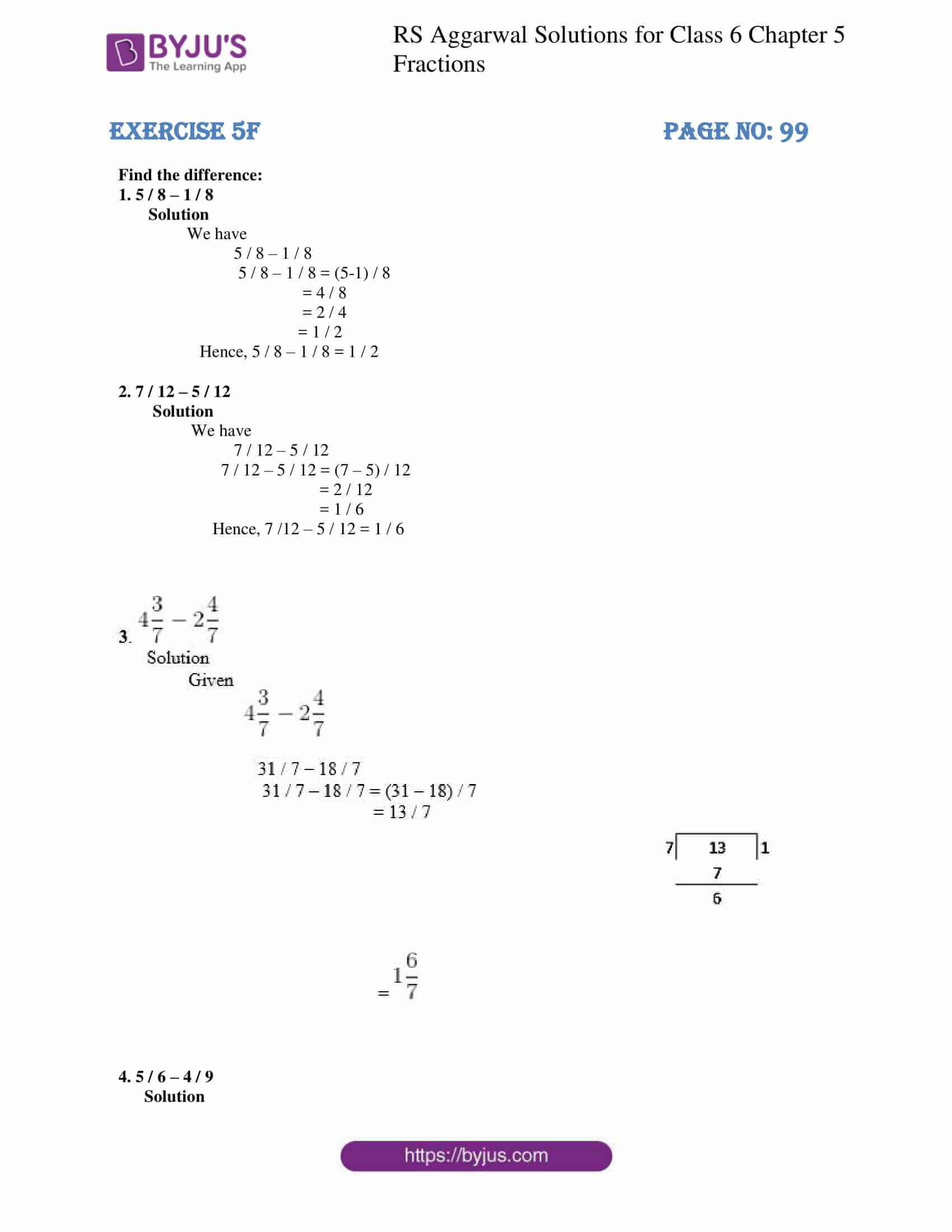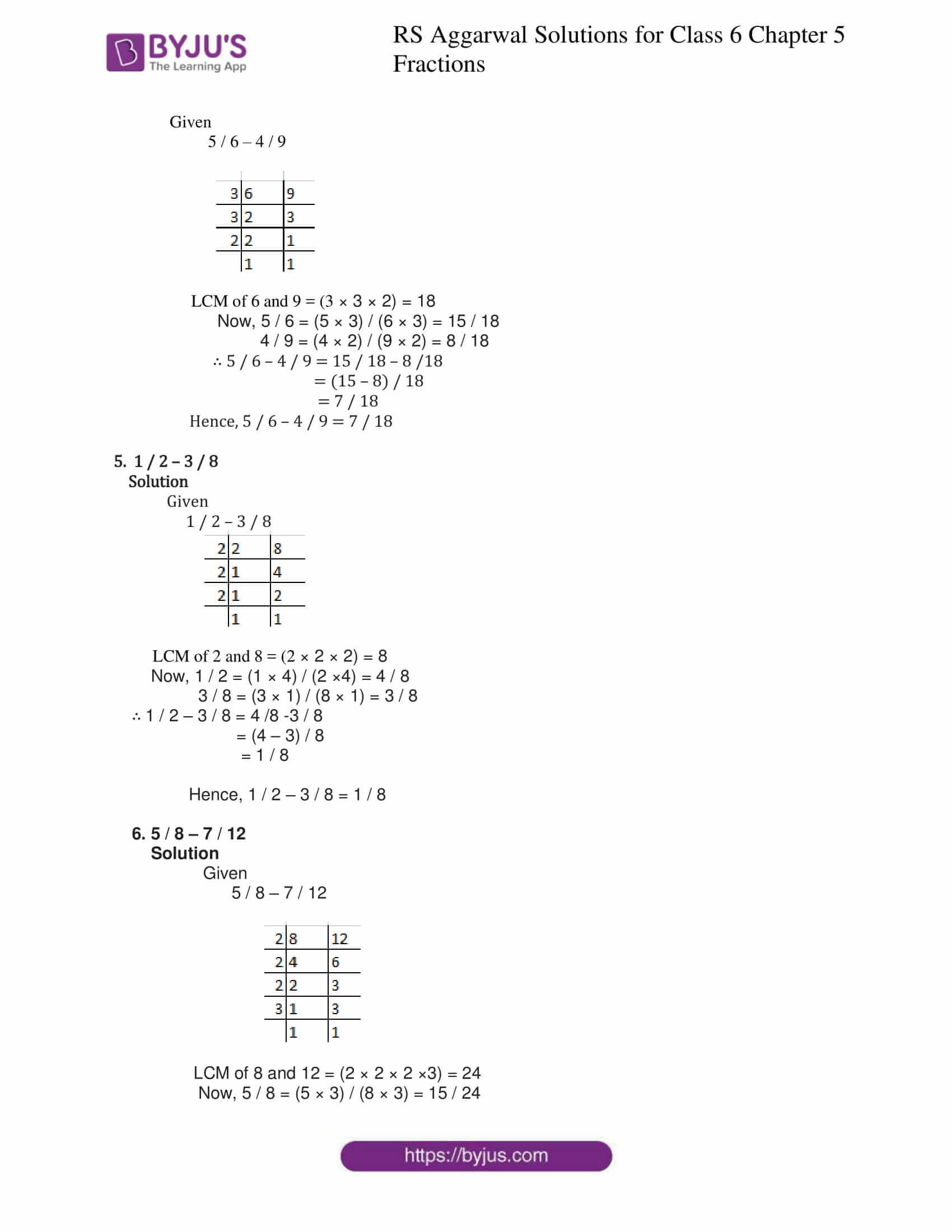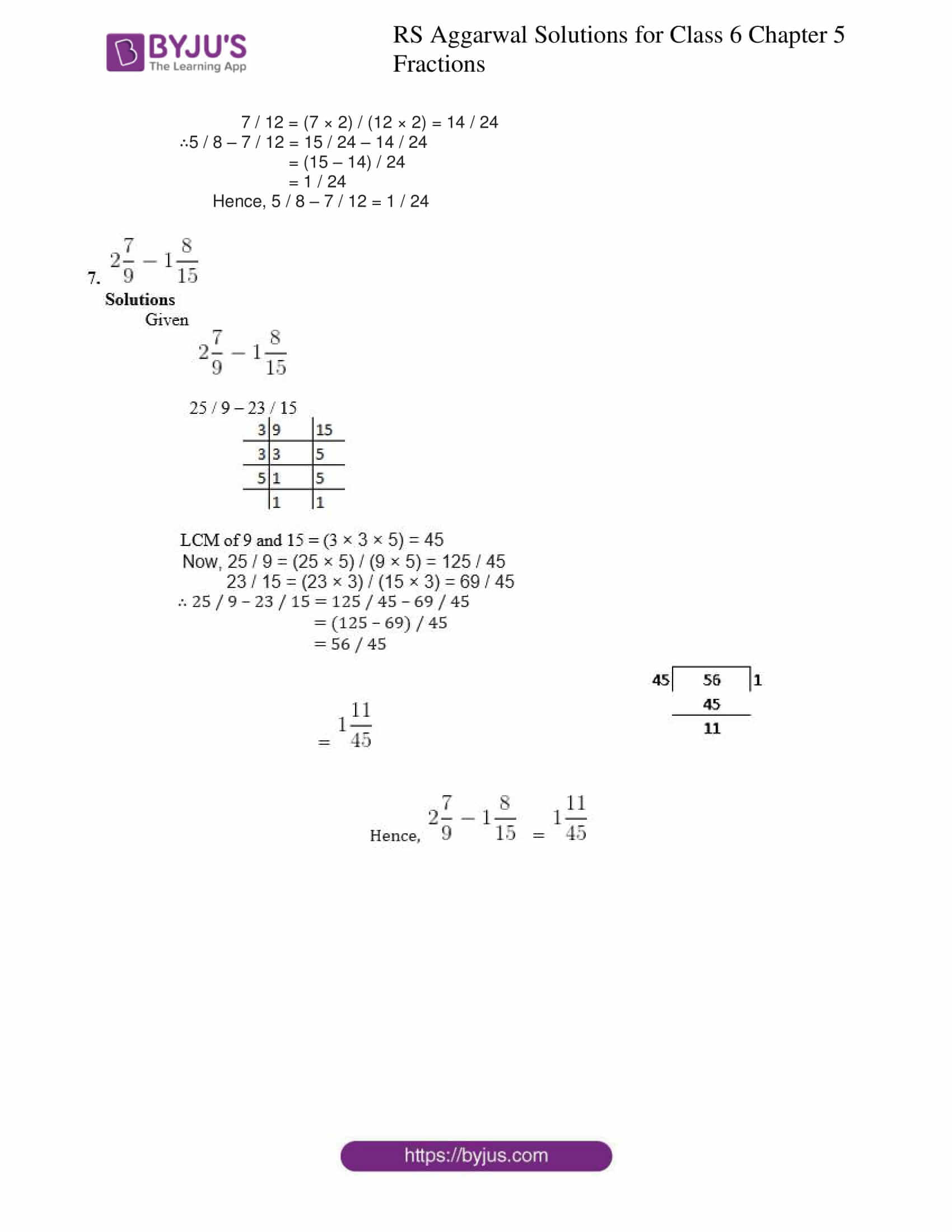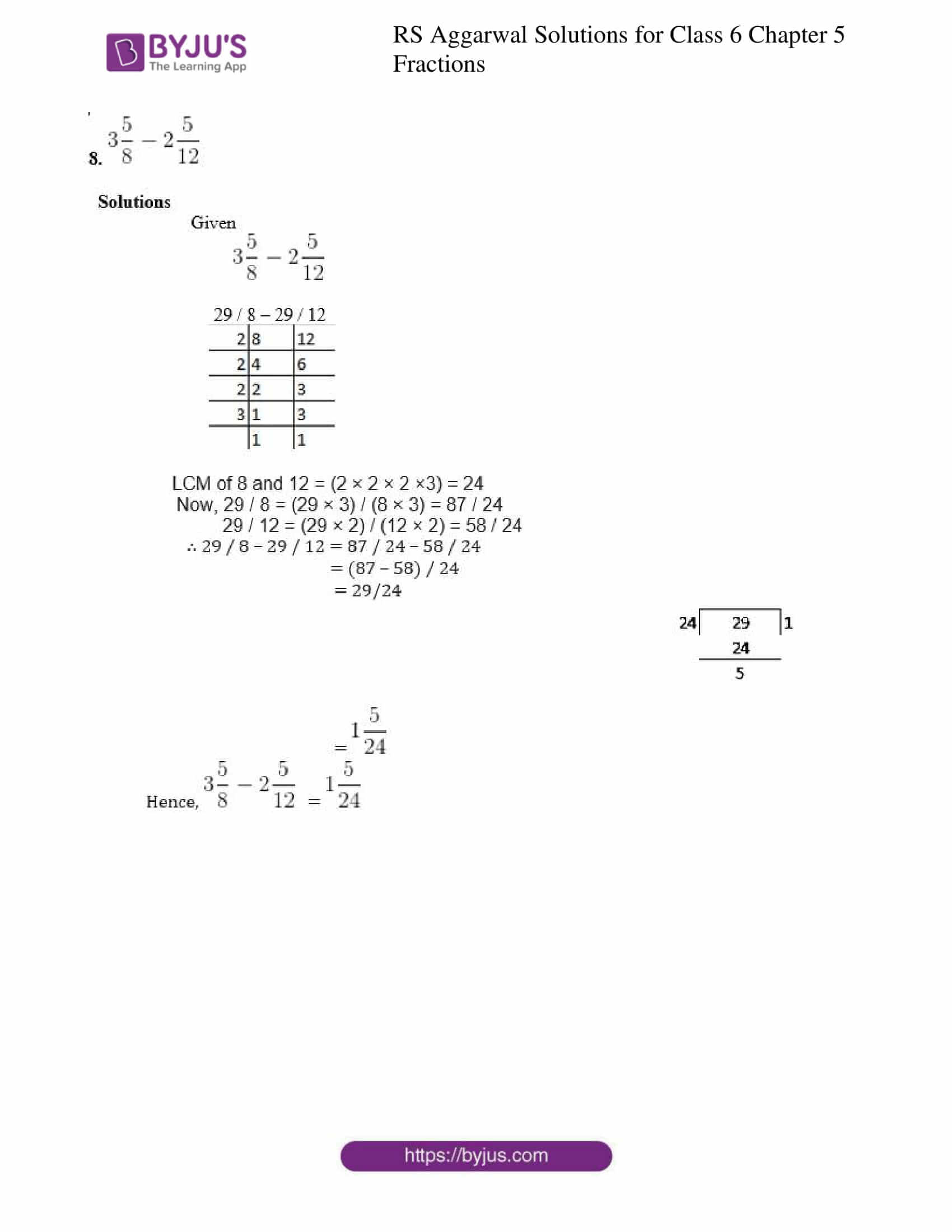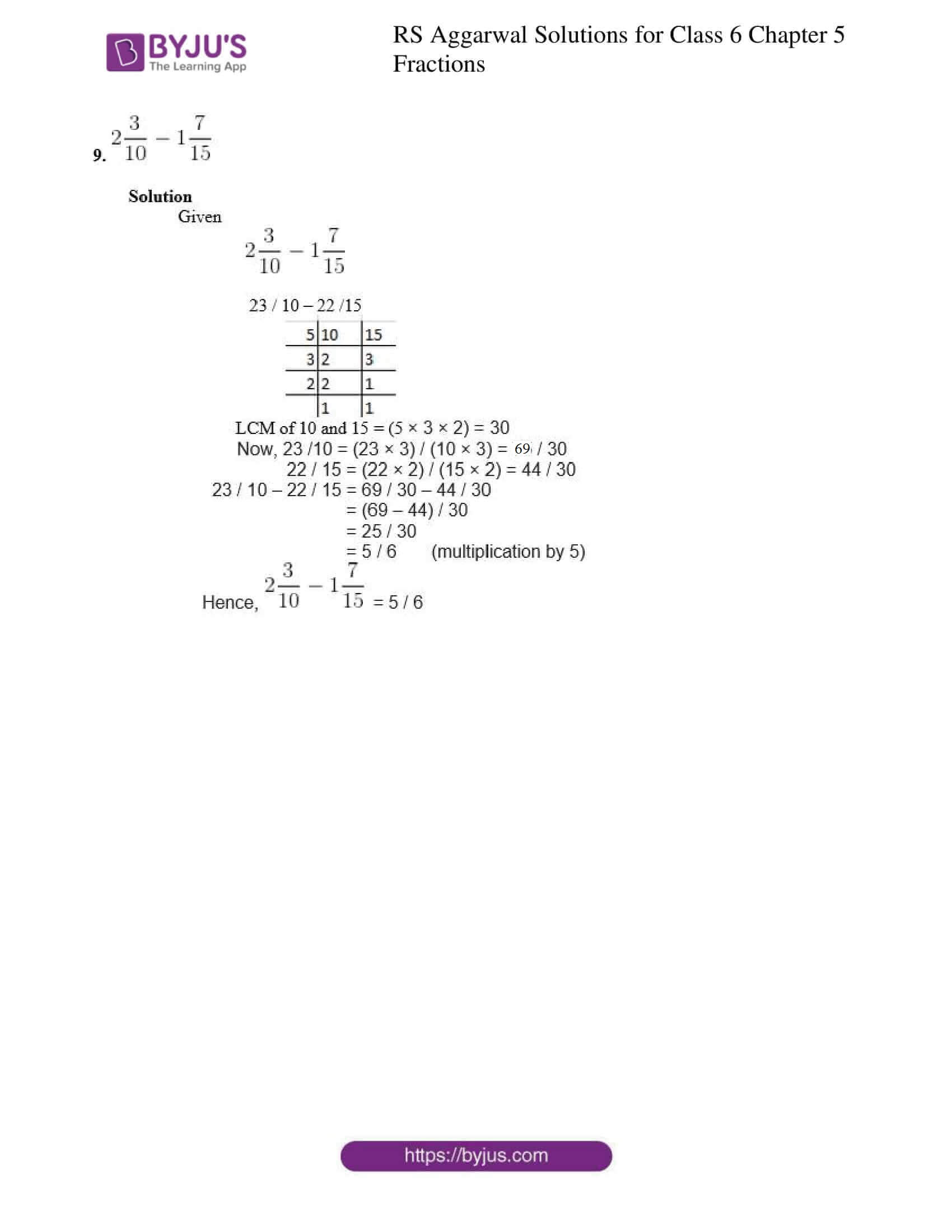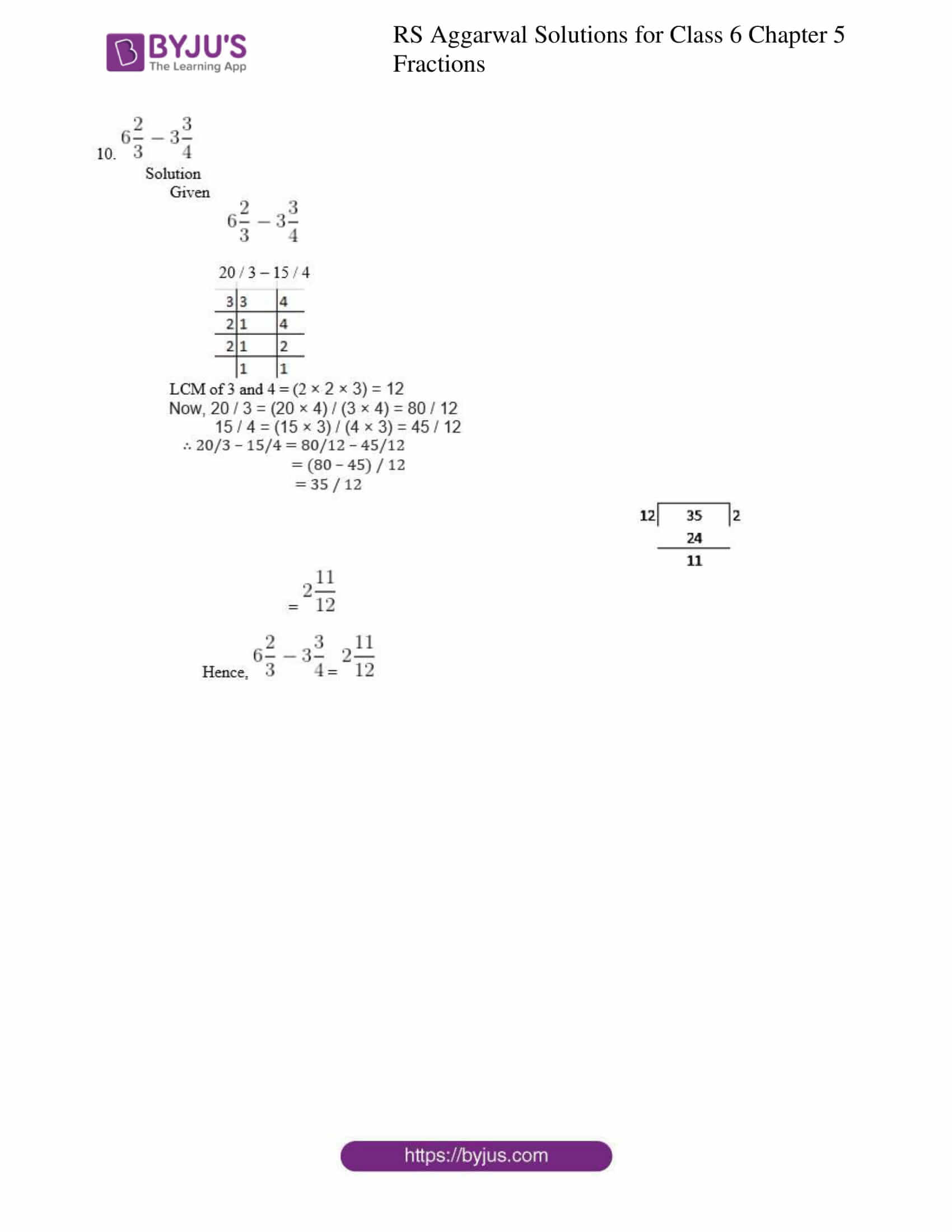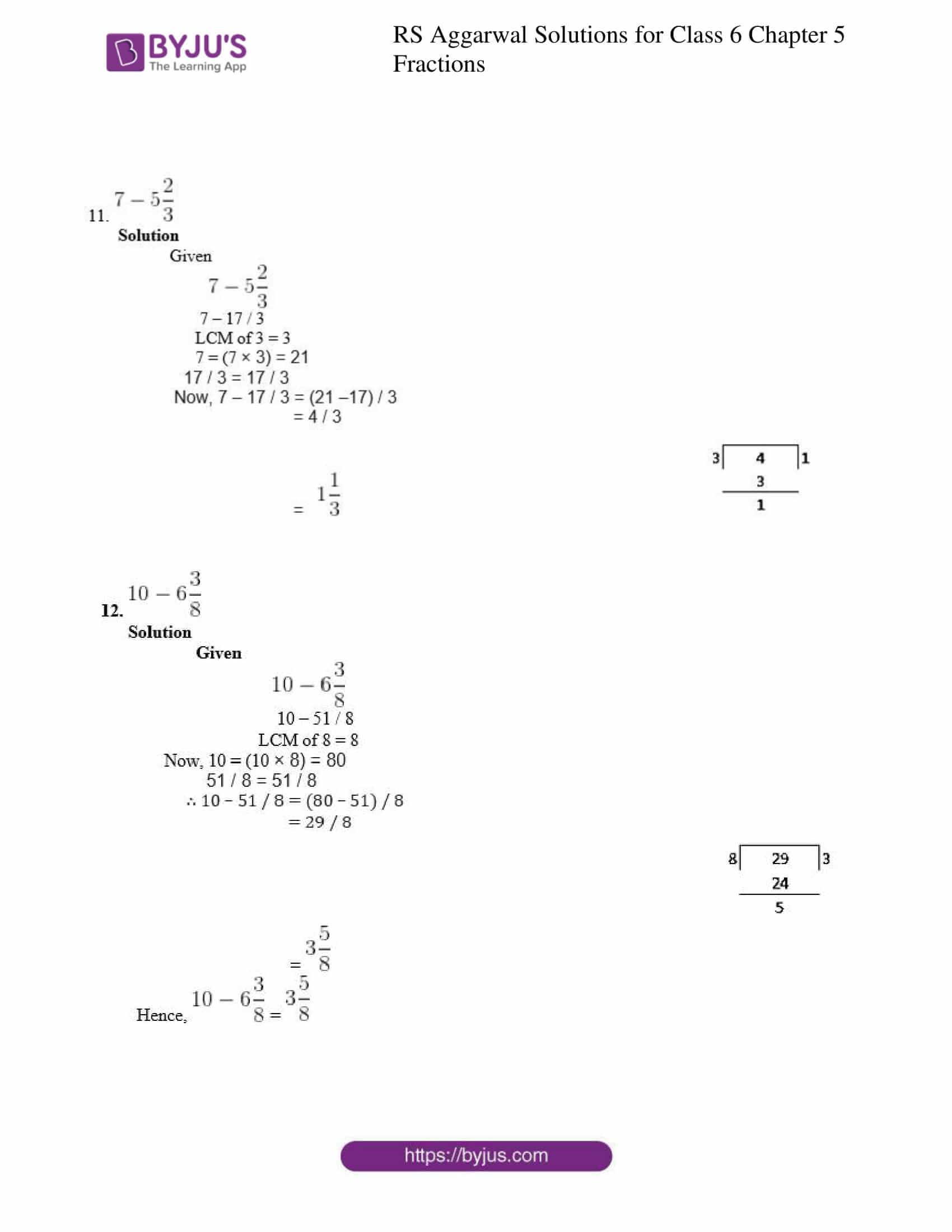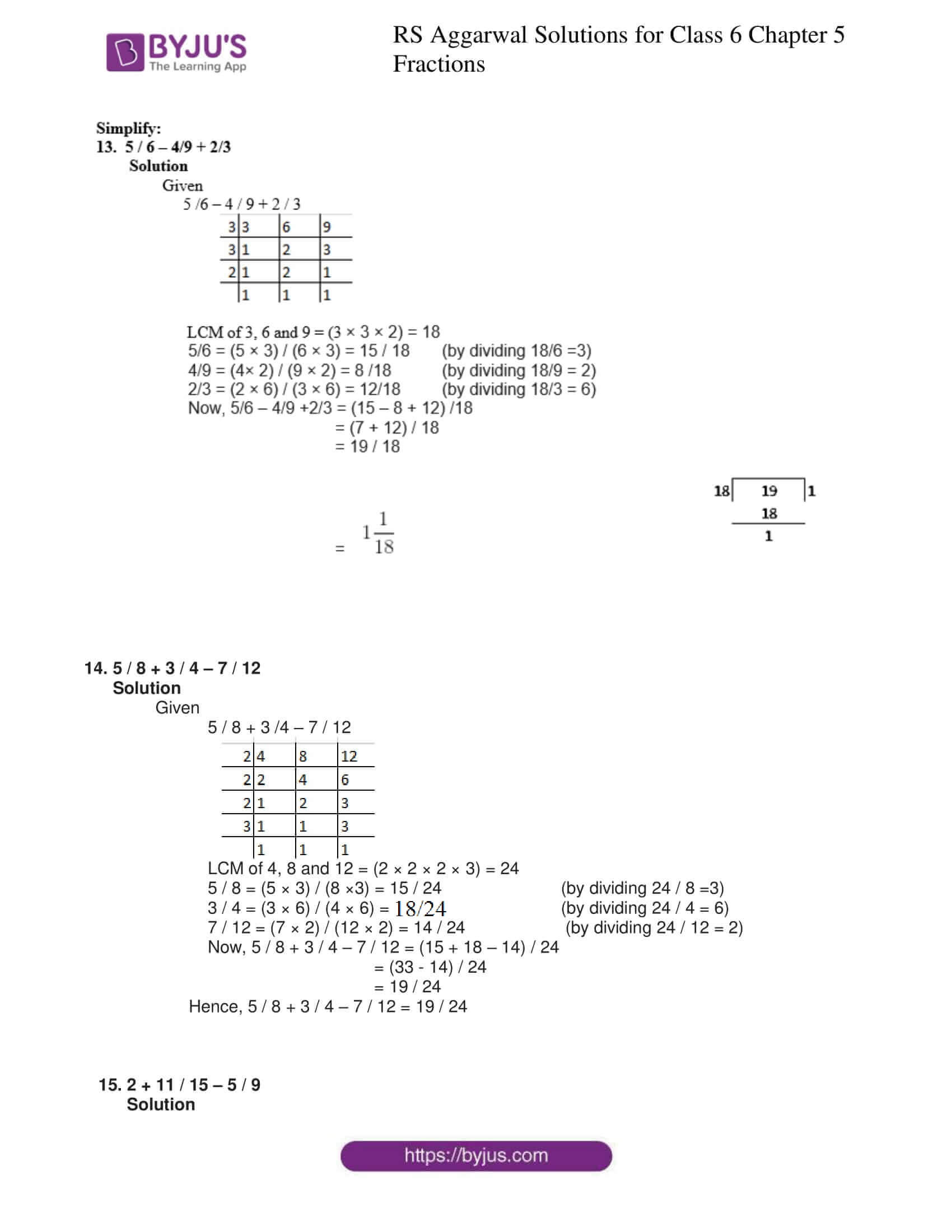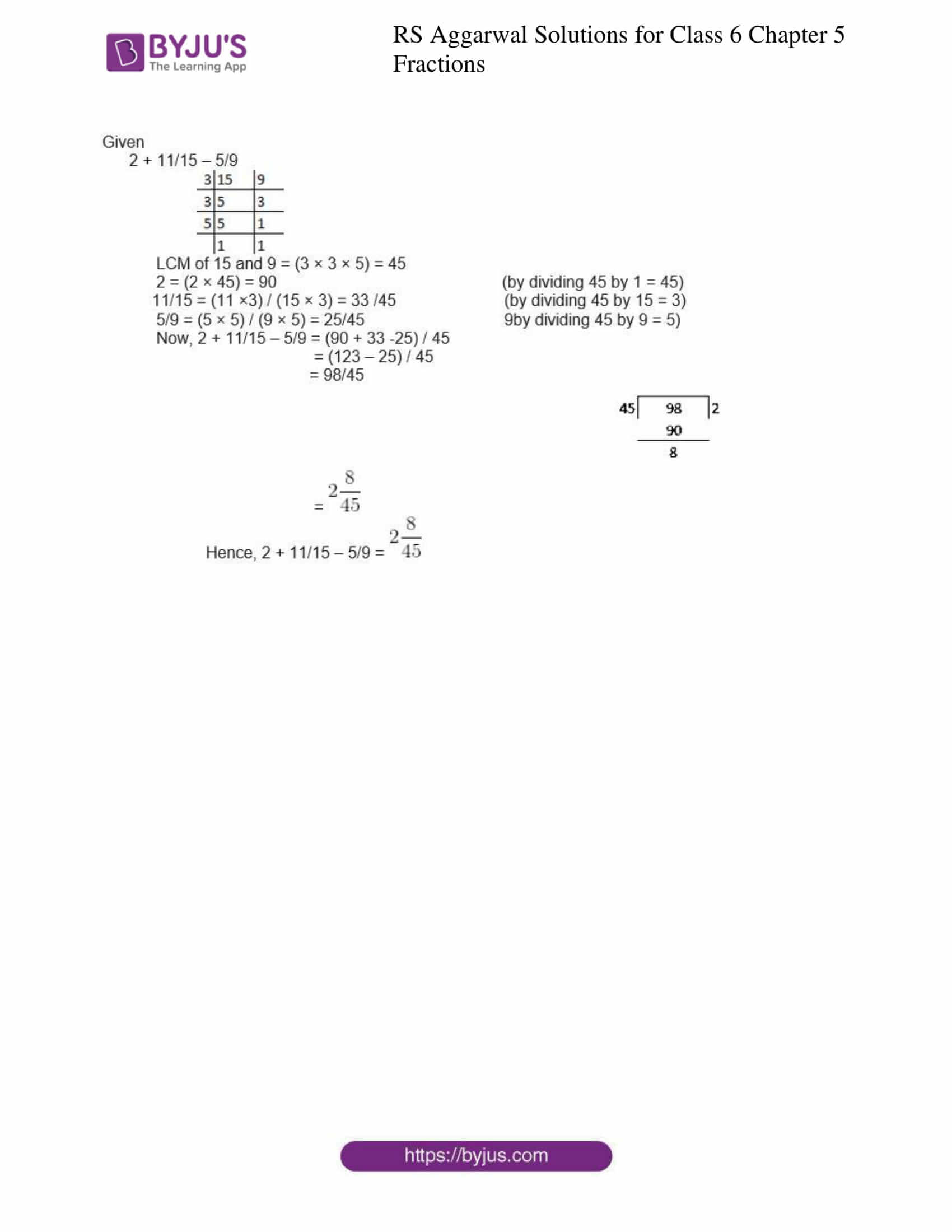### Access answers to Maths RS Aggarwal Solutions for Class 6 Chapter 5 Fractions Exercise 5F

Find the difference:

1. 5 / 8 – 1 / 8

Solution

We have

5 / 8 – 1 / 8

5 / 8 – 1 / 8 = (5-1) / 8

= 4 / 8

= 2 / 4

= 1 / 2

Hence, 5 / 8 – 1 / 8 = 1 / 2

2. 7 / 12 – 5 / 12

Solution

We have

7 / 12 – 5 / 12

7 / 12 – 5 / 12 = (7 – 5) / 12

= 2 / 12

= 1 / 6

Hence, 7 /12 – 5 / 12 = 1 / 6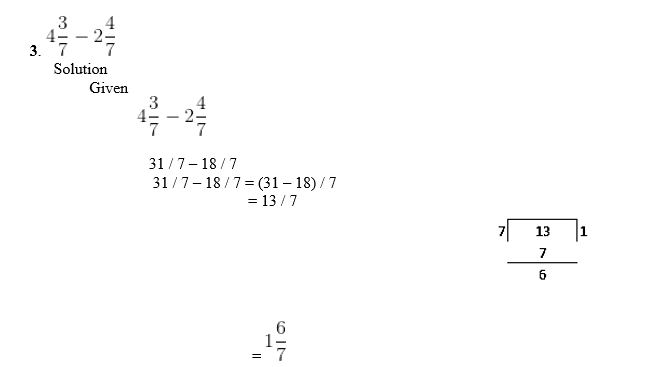4. 5 / 6 – 4 / 9

Solution

Given

5 / 6 – 4 / 9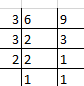LCM of 6 and 9 = (3 × 3 × 2) = 18

Now, 5 / 6 = (5 × 3) / (6 × 3) = 15 / 18

4 / 9 = (4 × 2) / (9 × 2) = 8 / 18

∴ 5 / 6 – 4 / 9 = 15 / 18 – 8 /18

= (15 – 8) / 18

= 7 / 18

Hence, 5 / 6 – 4 / 9 = 7 / 18

5. 1 / 2 – 3 / 8

Solution

Given

1 / 2 – 3 / 8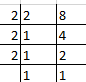LCM of 2 and 8 = (2 × 2 × 2) = 8

Now, 1 / 2 = (1 × 4) / (2 ×4) = 4 / 8

3 / 8 = (3 × 1) / (8 × 1) = 3 / 8

∴ 1 / 2 – 3 / 8 = 4 /8 -3 / 8

= (4 – 3) / 8

= 1 / 8

Hence, 1 / 2 – 3 / 8 = 1 / 8

6. 5 / 8 – 7 / 12

Solution

Given

5 / 8 – 7 / 12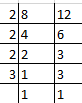LCM of 8 and 12 = (2 × 2 × 2 ×3) = 24

Now, 5 / 8 = (5 × 3) / (8 × 3) = 15 / 24

7 / 12 = (7 × 2) / (12 × 2) = 14 / 24

∴5 / 8 – 7 / 12 = 15 / 24 – 14 / 24

= (15 – 14) / 24

= 1 / 24

Hence, 5 / 8 – 7 / 12 = 1 / 24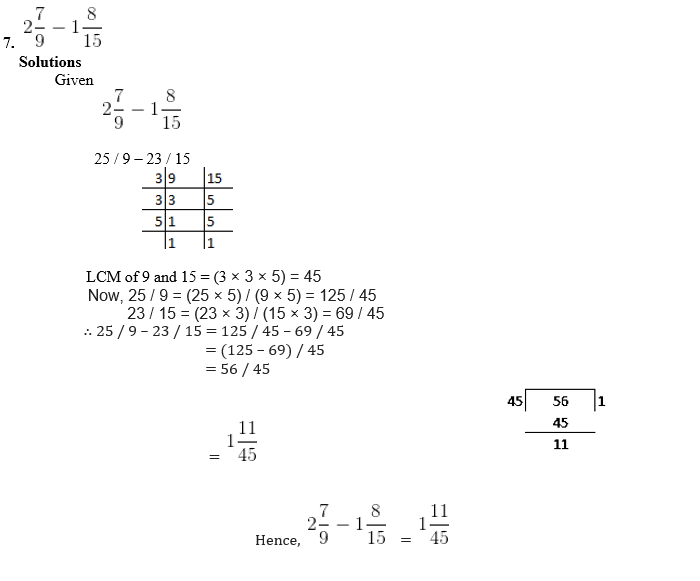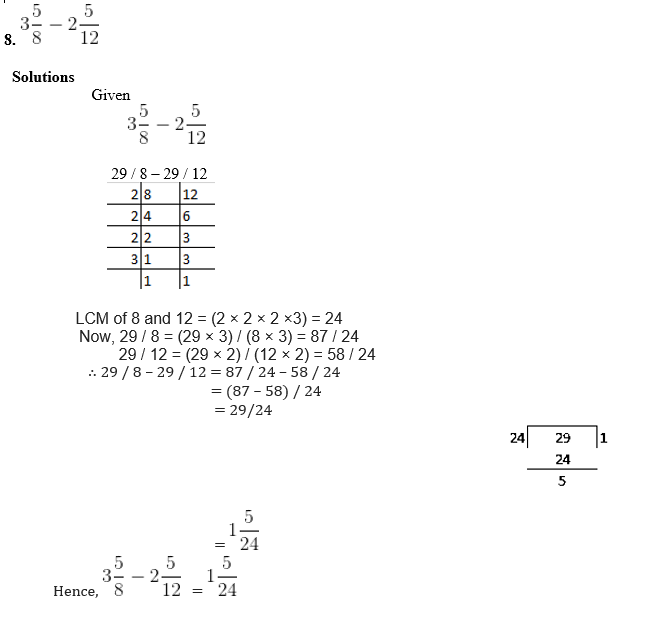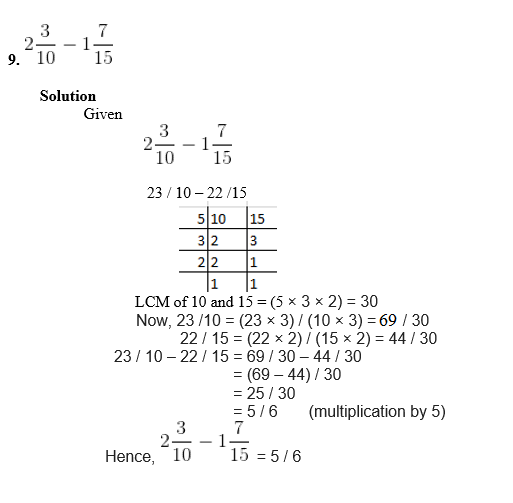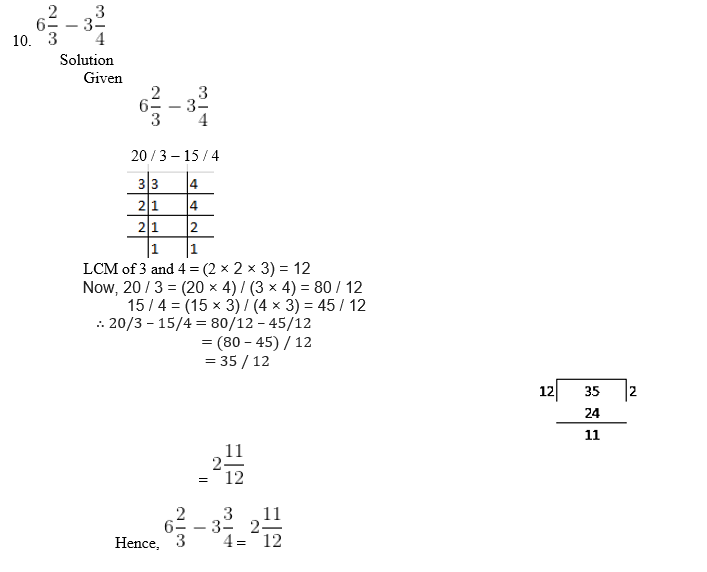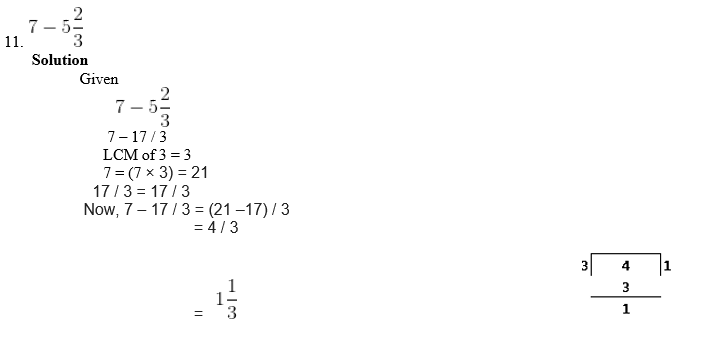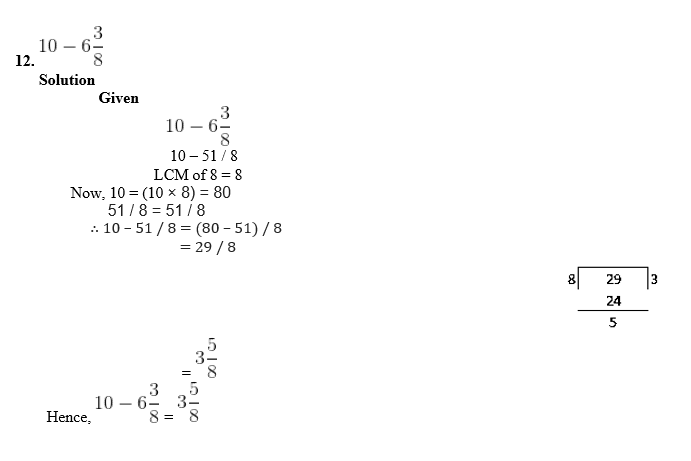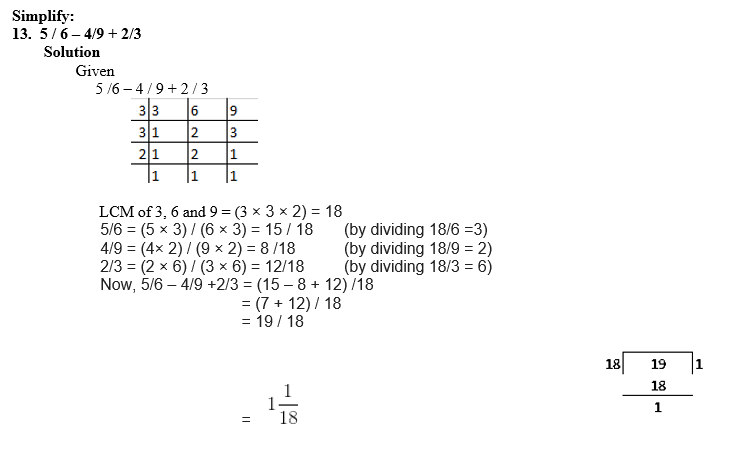14. 5 / 8 + 3 / 4 – 7 / 12

Solution

Given

5 / 8 + 3 /4 – 7 / 12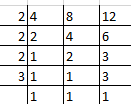LCM of 4, 8 and 12 = (2 × 2 × 2 × 3) = 24

5 / 8 = (5 × 3) / (8 ×3) = 15 / 24 (by dividing 24 / 8 =3)

3 / 4 = (3 × 6) / (4 × 6) = 18 / 24 (by dividing 24 / 4 = 6)

7 / 12 = (7 × 2) / (12 × 2) = 14 / 24 (by dividing 24 / 12 = 2)

Now, 5 / 8 + 3 / 4 – 7 / 12 = (15 + 18 – 14) / 24

= (33 – 14) / 24

= 19 / 24

Hence, 5 / 8 + 3 / 4 – 7 / 12 = 19 / 24

15. 2 + 11 / 15 – 5 / 9

Solution